+
Calculating z-Scores
Interpret Inferential Statistics
0
of 0 possible points

# Calculating z-Scores

Author: Sophia Tutorial
##### Description:

Calculate a z-score from a given mean, standard deviation, and x-value.

(more)

Sophia’s self-paced online courses are a great way to save time and money as you earn credits eligible for transfer to many different colleges and universities.*

No credit card required

37 Sophia partners guarantee credit transfer.

299 Institutions have accepted or given pre-approval for credit transfer.

* The American Council on Education's College Credit Recommendation Service (ACE Credit®) has evaluated and recommended college credit for 32 of Sophia’s online courses. Many different colleges and universities consider ACE CREDIT recommendations in determining the applicability to their course and degree programs.

Tutorial
what's covered
This lesson discusses calculating z-scores. You will understand what a z-score actually is, and be able to determine one if you were given a mean and a standard deviation of a normally distributed variable. This lesson covers:
1. Normal Distribution and z-Scores
2. Properties of a z-Distribution Graph
3. Calculating a z-Score

## 1. Normal Distribution and z-Scores

Remember from previous lessons that normal distributions have bell-shaped curves.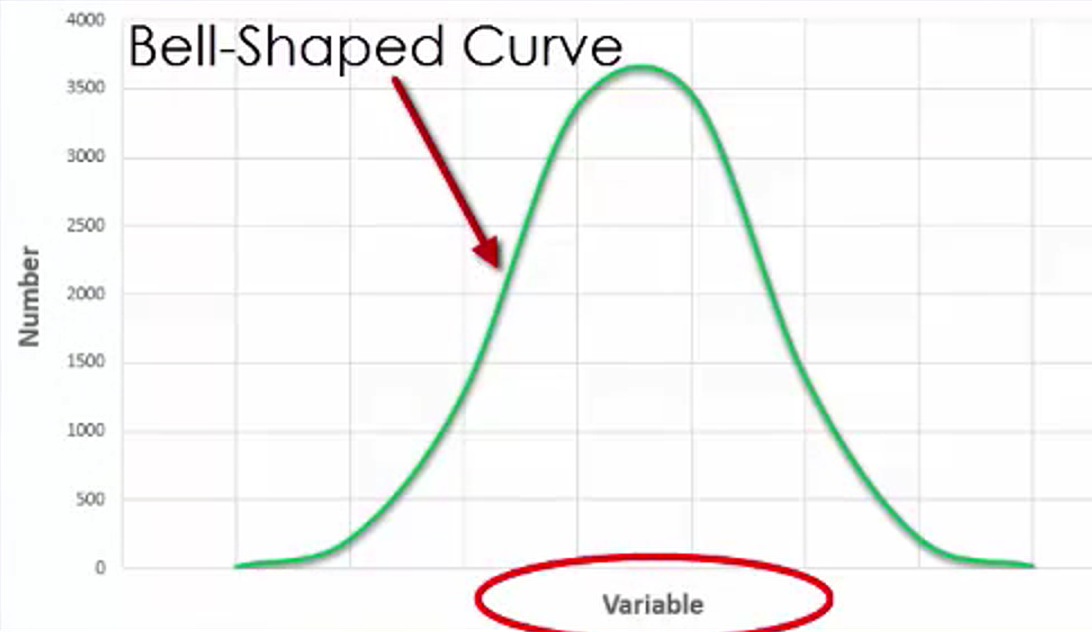This curve represents the values of a variable and how they are distributed across a range. In a normal distribution, the mean is the center of the distribution. The standard deviation represents how spread out the observations are across the distribution.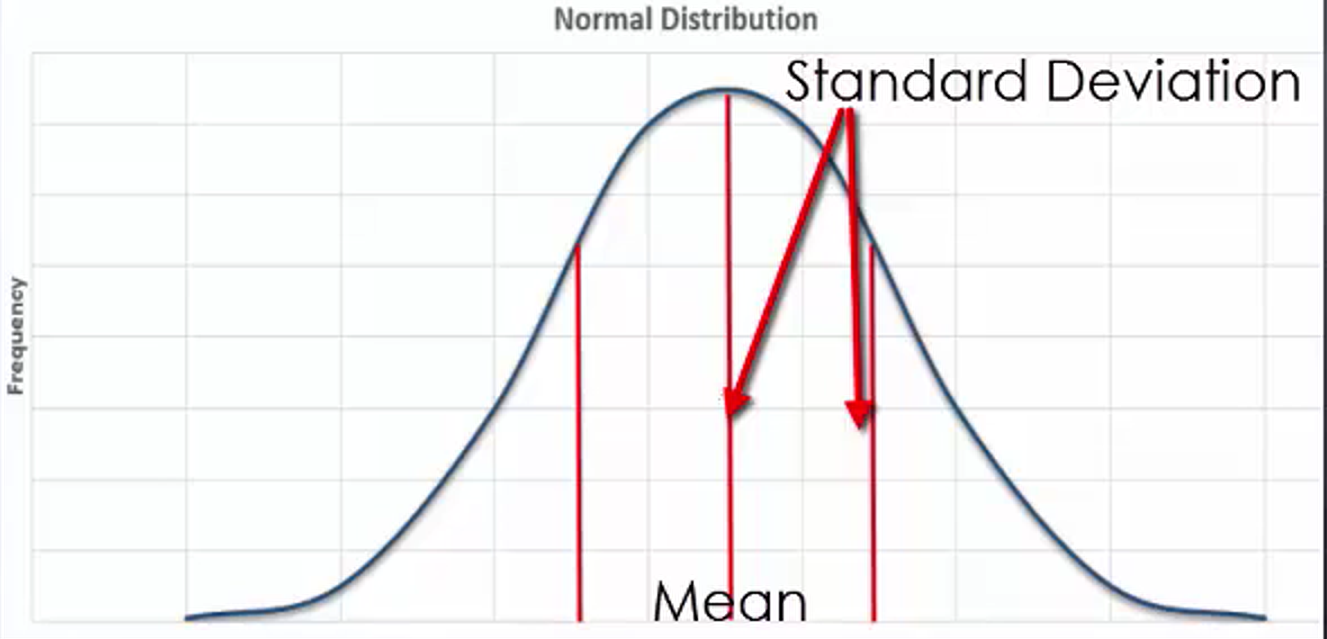The key reason we use a standard normal distribution is that doing so enables us to easily determine a probability associated with a specific value regarding any normal distribution.

In any standard normal distribution, the mean is always equal to 0 and the standard deviation is always equal to 1. A z-score is what we use to represent the values of the mean and standard deviation when using a standard normal distribution, with a z-score of 0 being equal to the mean and any other value being equivalent to how many standard deviations away from the mean a value lies.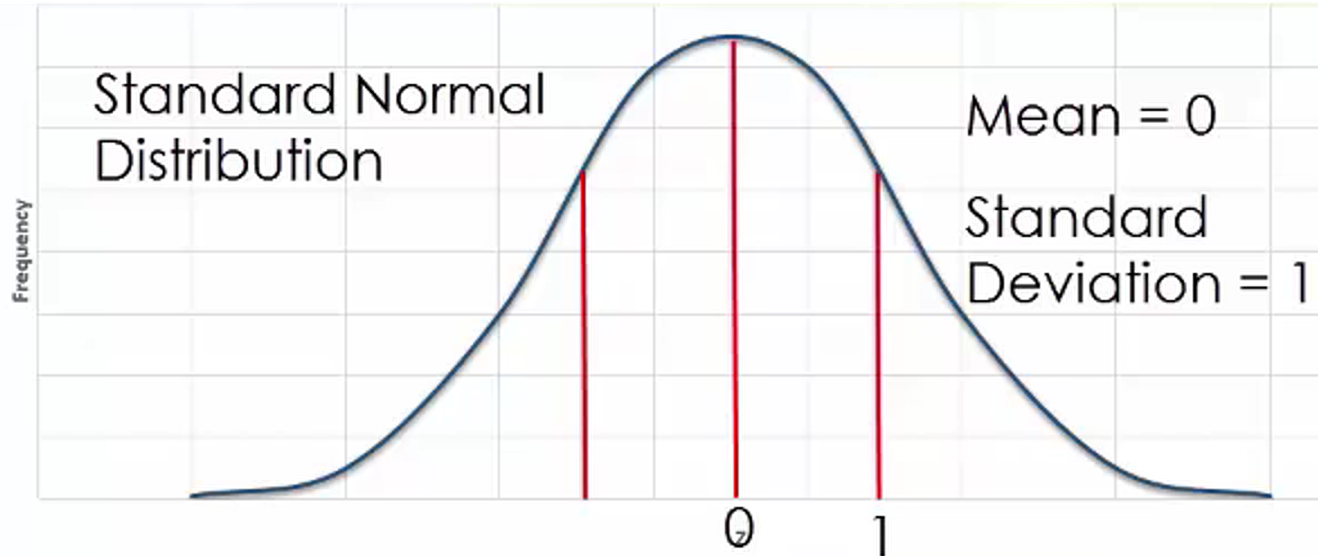The nice thing about a standard normal distribution is that it can be applied to any normal distribution, so long as you know the mean and standard deviation specific to that distribution. You do this by using the formula:

formula
Z-Score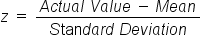With a normal distribution, as long as you know the mean and the standard deviation, you can determine a z-score. When using the z-score formula, you must subtract the mean from the specific value of interest prior to dividing by the standard deviation.

Remember that population data comes from a larger realm of all values of a specific variable while sample data comes from a smaller number of observations that are chosen by the researcher. While the mean and the standard deviation can be from either a sample or population when applied to the z-score formula, in this specific instance we will only look at data that comes from a sample.

IN CONTEXT

There are a variety of different situations in which you might want to convert data into a z-score. One of these could be test scores such as the ACT or the SAT.

Or, suppose you want to measure the size of a particular species specimen. Looking at z-scores would be helpful if you’re interested in whether or not it happens to be a relatively large specimen or a relatively small one.

Looking at economics, you could get a sense of the distribution of household income and the probabilities associated with whether a particular household falls in a relatively high or low range in this regard. By converting values of a variable into z-scores, you are able to make certain that you adhere to a standard process for analyzing data.

term to know

Z-Score
Indicates how many standard deviations away from the mean a value lies

## 2. Properties of a z-Distribution Graph

This graph represents what a z-distribution looks like.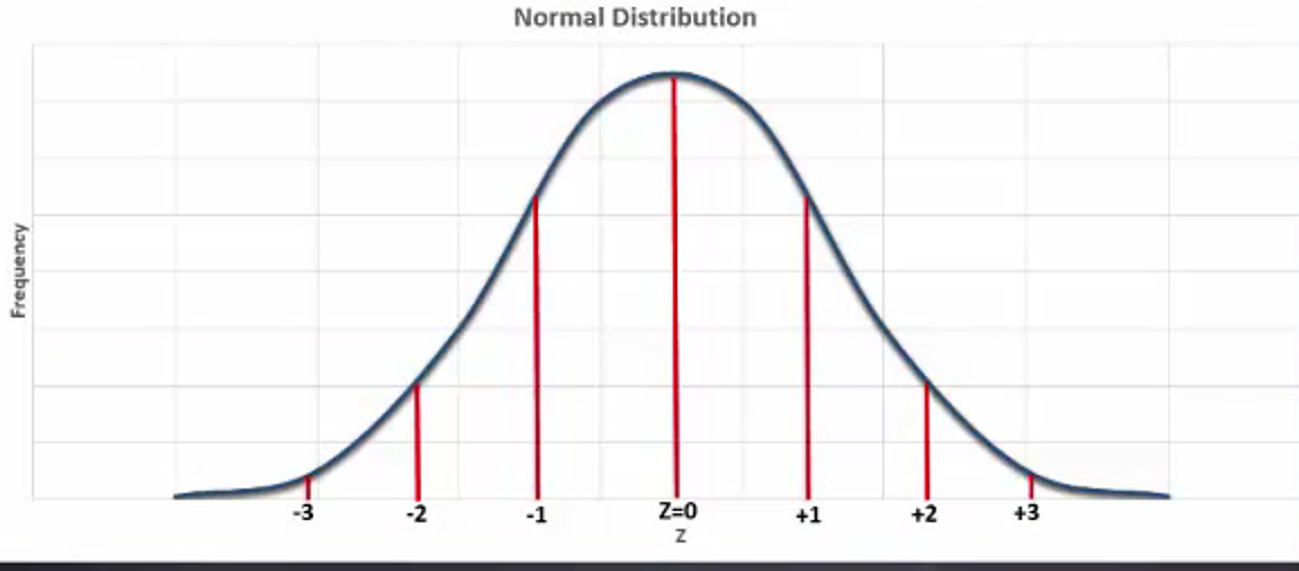Notice that you have individual numbers that represent how many standard deviations away from the mean a particular point lies. On the horizontal axis, one unit is equal to one standard deviation of the z-distribution.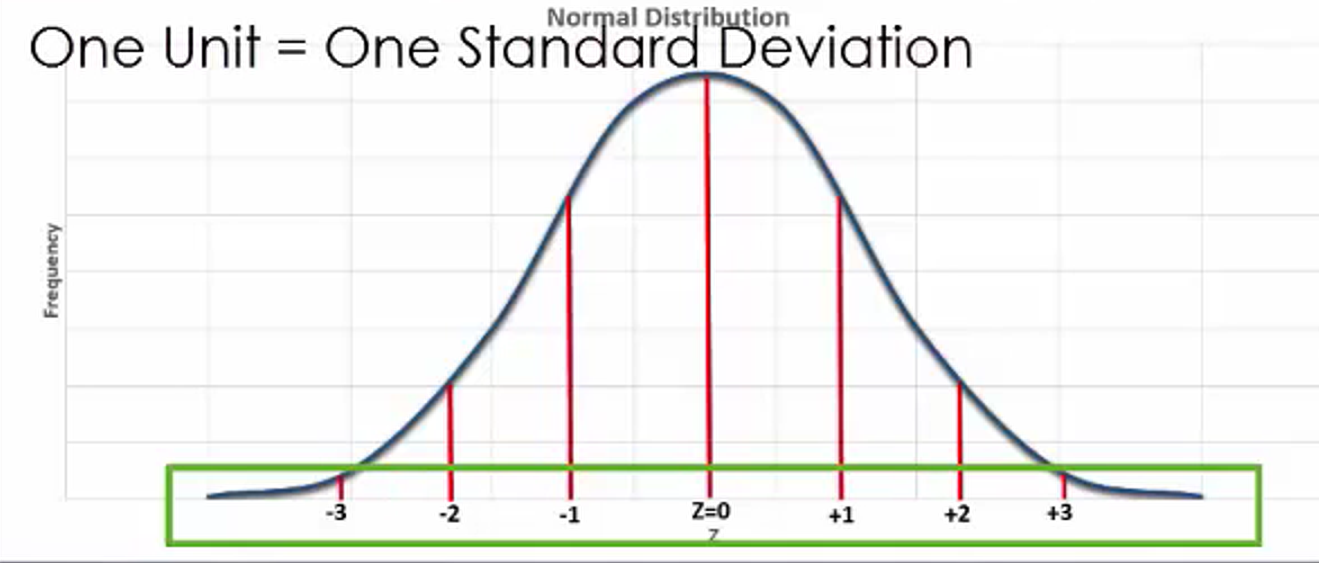When you look at graphs of a normal distribution, it’s helpful to think of the z-score as illustrating how many standard deviations a value lies above the mean, if the z-score happens to be positive, or below the mean, if the z-score happens to be negative.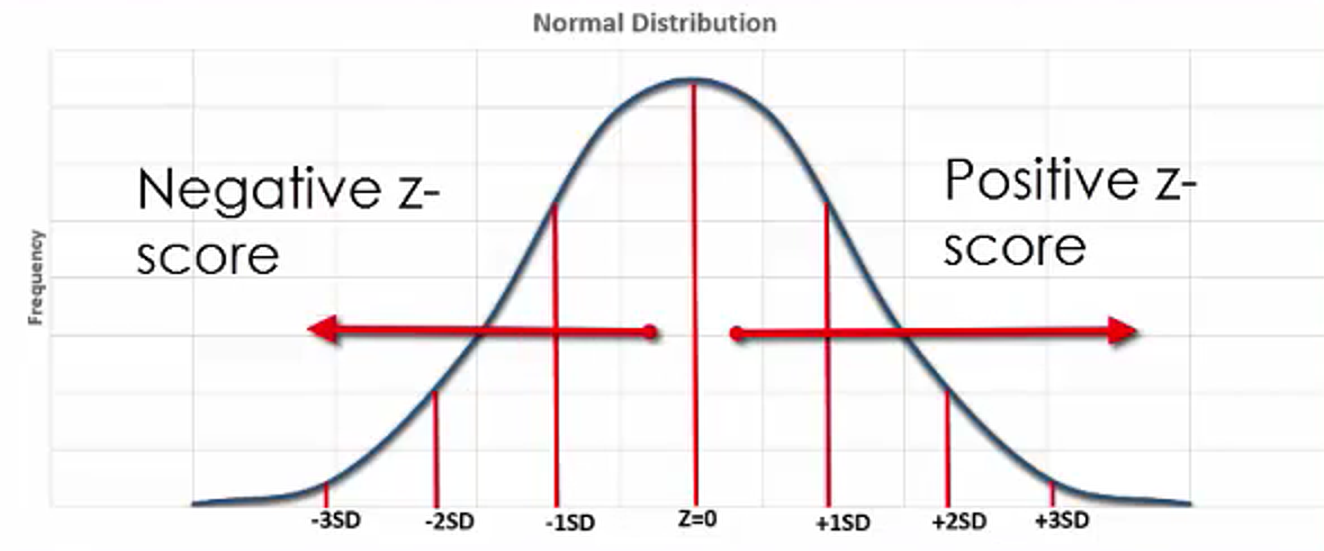## 3. Calculating a z-Score

Suppose you were to look at the morning commute times for Americans. They are normally distributed with a mean of 25 minutes and a standard deviation of four minutes. The graph here illustrates this distribution, as well as where the mean is located and the points that are one standard deviation to the left and right of the mean.The z-score for mean is 0: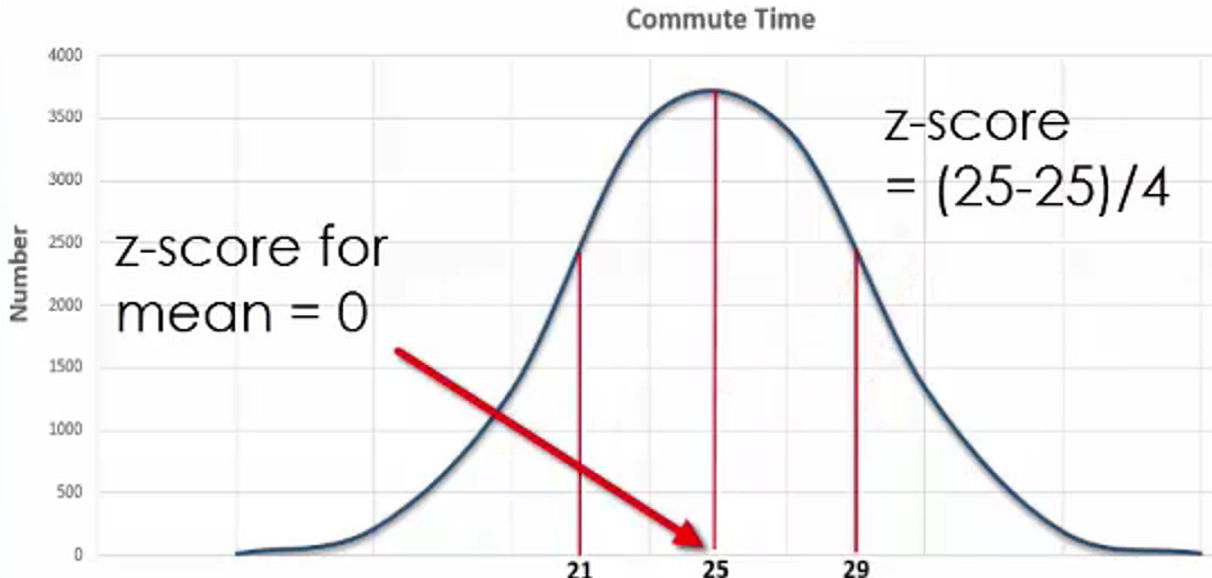The z-score for 21 minutes is -1: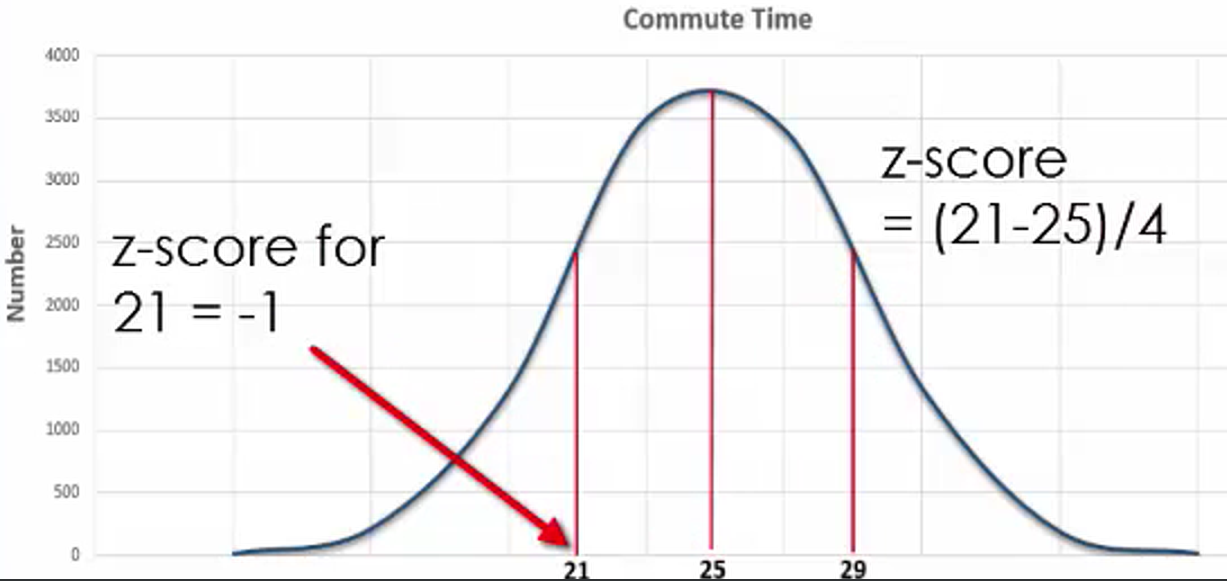The z-score for 29 minutes is 1: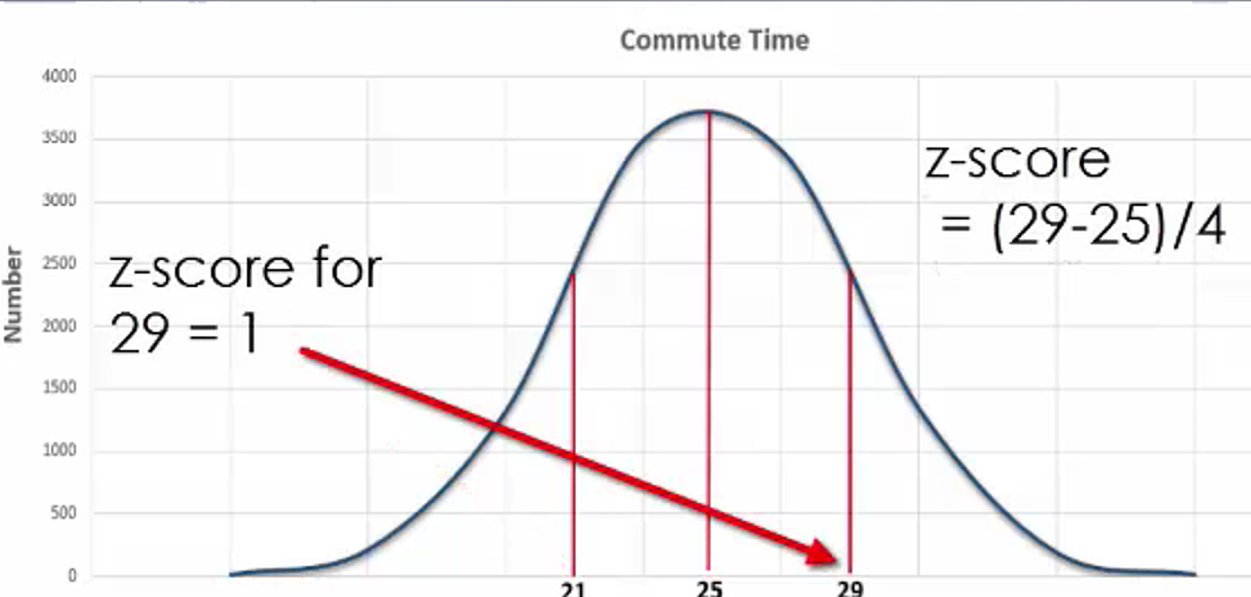Each of these values would be either one standard deviation to the left of the mean or one standard deviation to the right of the mean. If we add values to the graph corresponding to other quantities of standard deviations away from the mean, we can see the related z-scores.

13 minutes has a z-score of -3: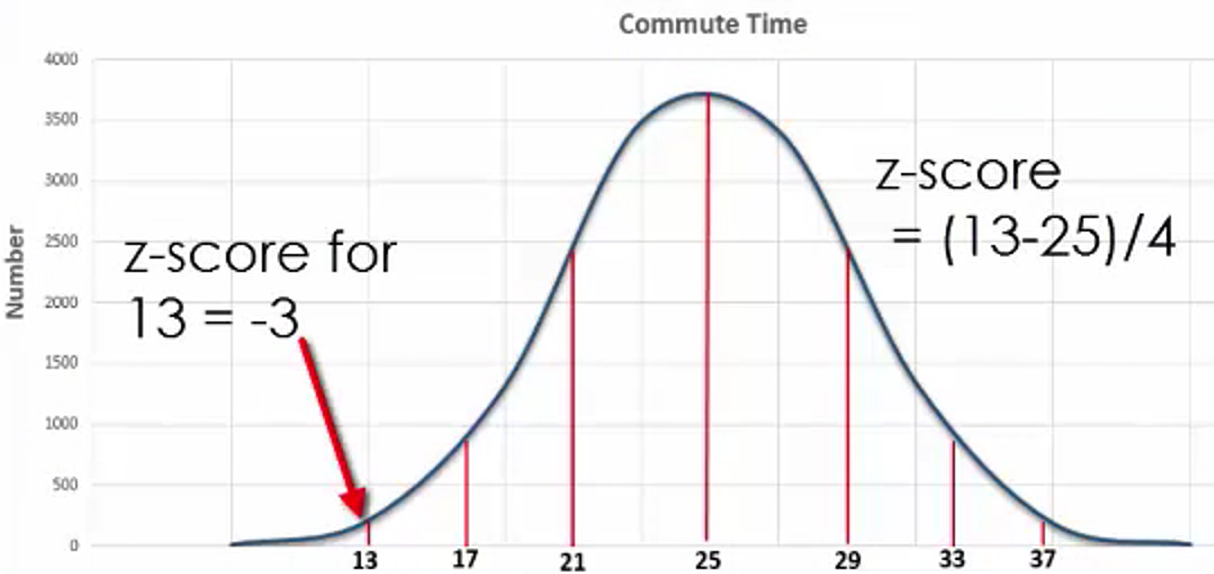17 minutes has a z-score of -2: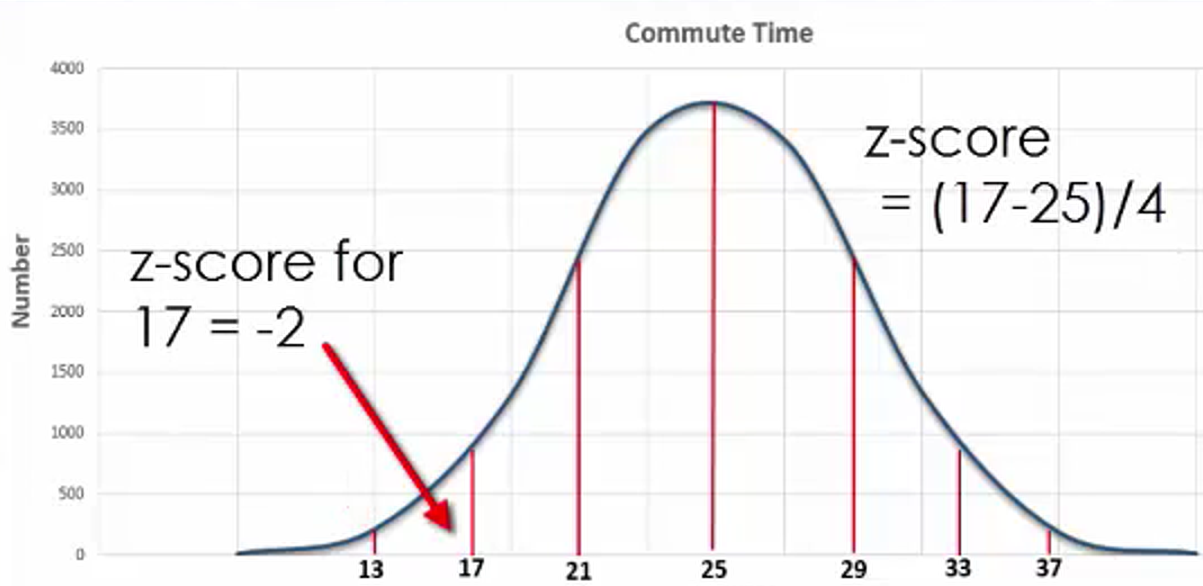33 minutes has a z-score of 2: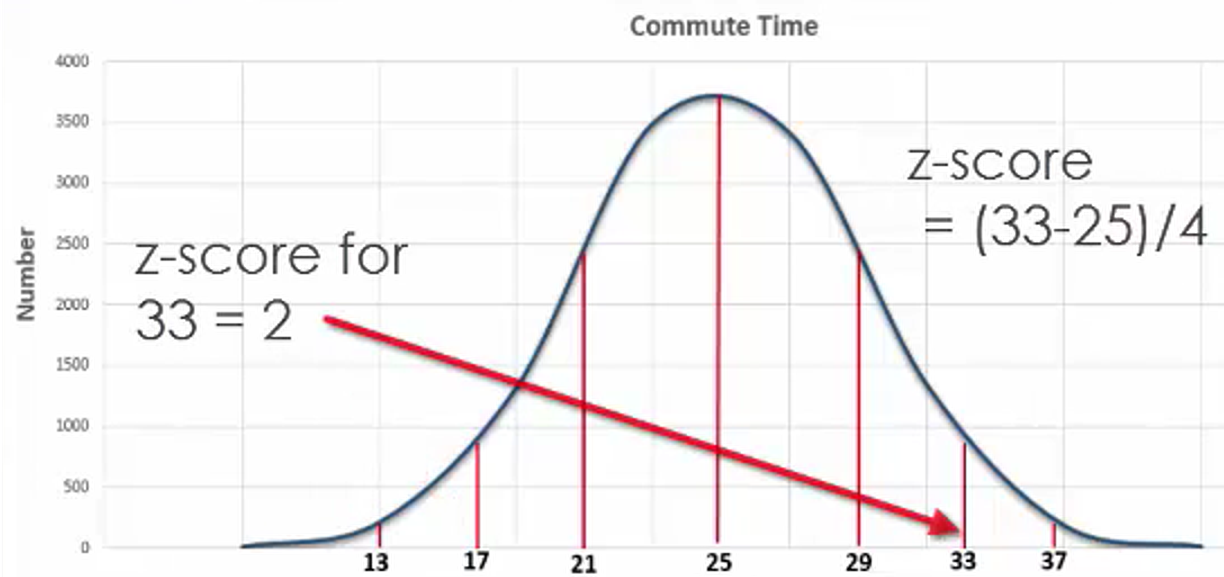37 minutes has a z-score of 3: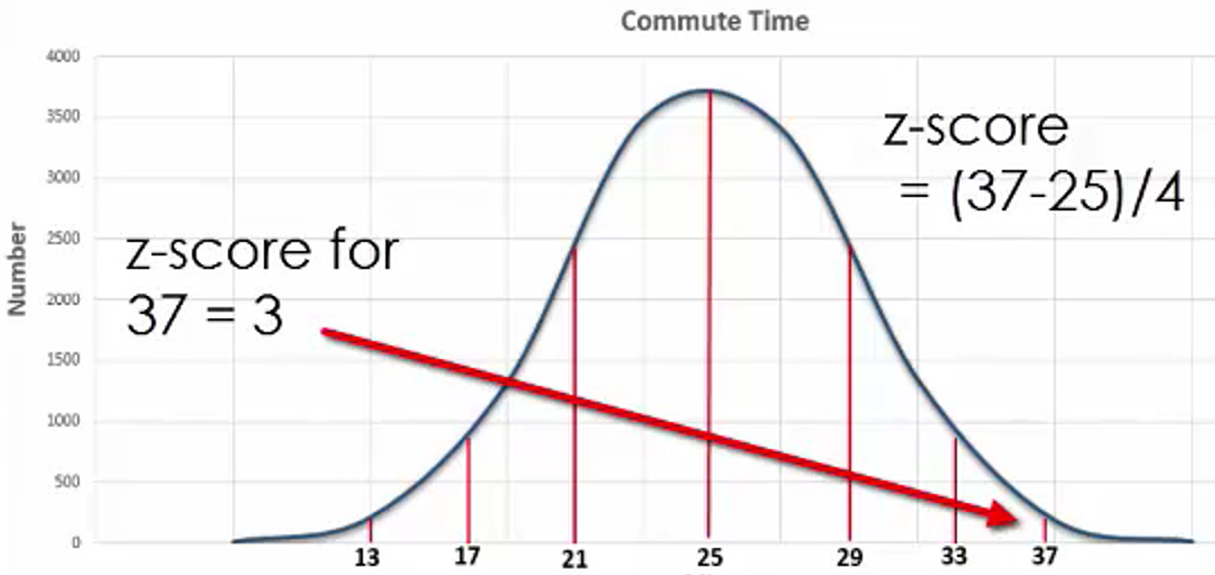There are often values of a variable that don’t directly correspond to a whole number of standard deviations away from the mean.

try it
What would the z-score for someone with a commute time of 16 minutes be?

The z-score would be -2.25.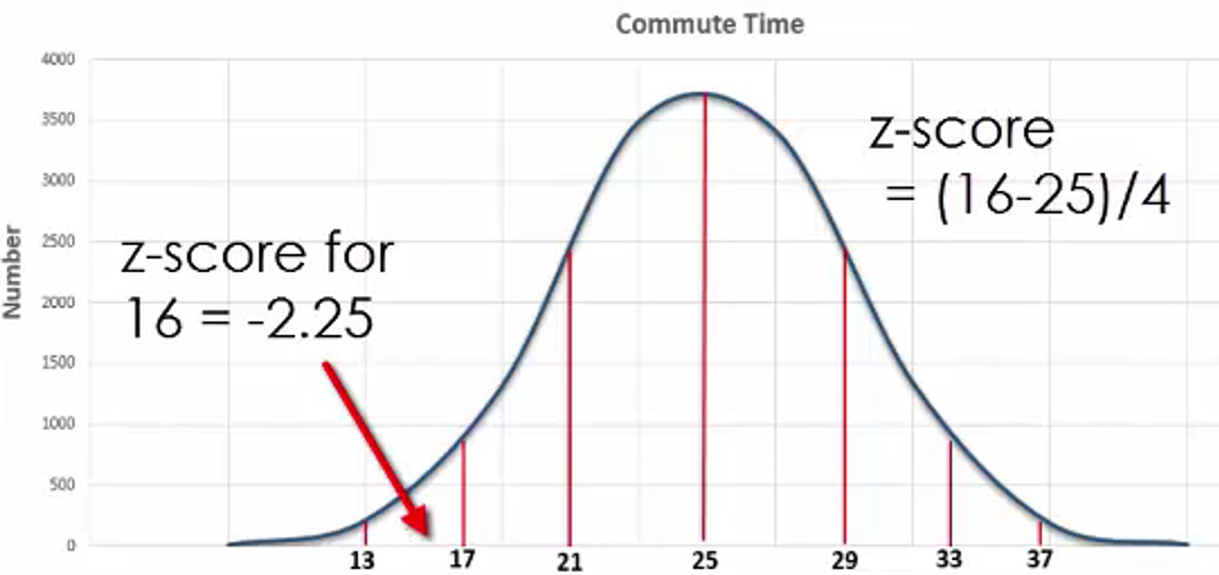What would the z-score be for someone with a commute time of 39 minutes?

The z-score would be 3.5.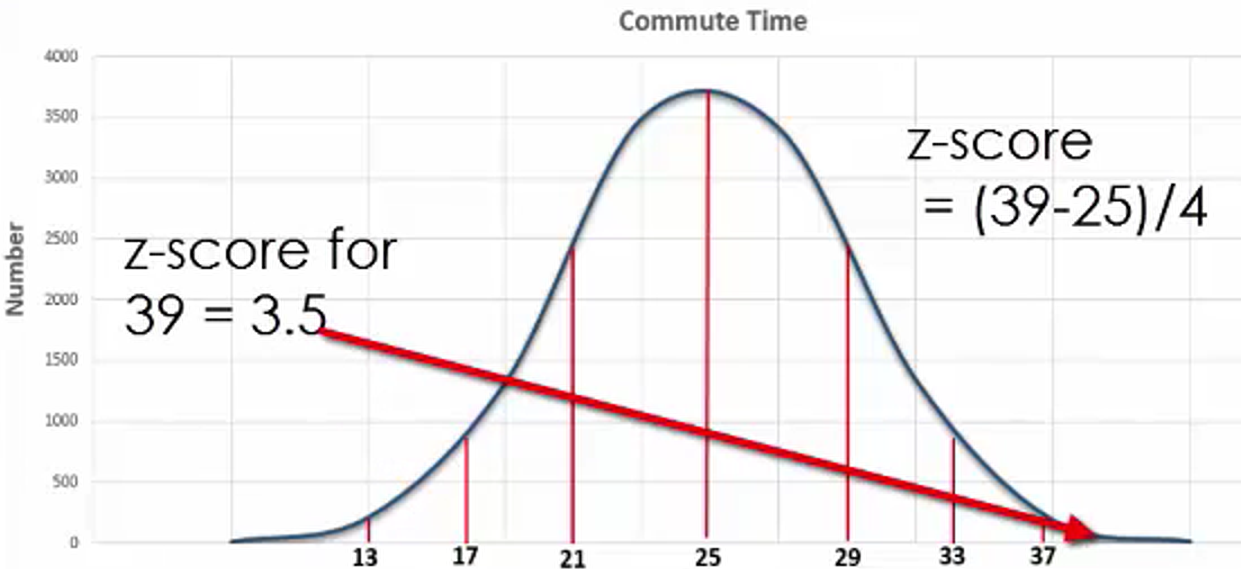Knowing a z-score can help you determine how likely it is that an event will occur.

summary
This lesson reviewed the relationship between normal distributions and z-scores. You should now be able to determine a z-score given a mean and standard deviation of a normally distributed variable. You also looked at the properties of a z-distribution graph. The z-score represents standard distributions above and below the mean. You also practiced calculating a z-score using an example of commute times.

Source: This work is adapted from Sophia author Dan Laub.

Terms to Know
Z-Score

Indicates how many standard deviations away from the mean a value lies

Formulas to Know
Z-ScoreRating